Updating search results...

# 13 Results

View
Selected filters:
• function-notationUnrestricted Use
CC BY
Rating
0.0 stars

The problem statement describes a changing algae population as reported by the Maryland Department of Natural Resources. In part (a), students are expected to build an exponential function modeling algae concentration from the description given of the relationship between concentrations in cells/ml and days of rapid growth (F-LE.2).

Subject:
Functions
Mathematics
Material Type:
Activity/Lab
Provider:
Illustrative Mathematics
Provider Set:
Illustrative Mathematics
Author:
Illustrative Mathematics
05/01/2012Unrestricted Use
CC BY
Rating
0.0 stars

This simple task assesses whether students can interpret function notation. The four parts of the task provide a logical progression of exercises for advancing understanding of function notation and how to interpret it in terms of a given context.

Subject:
Functions
Mathematics
Material Type:
Activity/Lab
Provider:
Illustrative Mathematics
Provider Set:
Illustrative Mathematics
Author:
Illustrative Mathematics
05/01/2012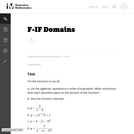Unrestricted Use
CC BY
Rating
0.0 stars

The purpose of this task to help students think about an expression for a function as built up out of simple operations on the variable, and understand the domain in terms of values for which each operation is invalid (e.g., dividing by zero or taking the square root of a negative number).

Subject:
Functions
Mathematics
Material Type:
Activity/Lab
Provider:
Illustrative Mathematics
Provider Set:
Illustrative Mathematics
Author:
Illustrative Mathematics
05/01/2012Unrestricted Use
CC BY
Rating
0.0 stars

The purpose of this task is to investigate the meaning of the definition of function in a real-world context where the question of whether there is more than one output for a given input arises naturally. In more advanced courses this task could be used to investigate the question of whether a function has an inverse.

Subject:
Functions
Mathematics
Material Type:
Activity/Lab
Provider:
Illustrative Mathematics
Provider Set:
Illustrative Mathematics
Author:
Illustrative Mathematics
05/01/2012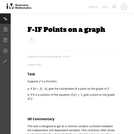Unrestricted Use
CC BY
Rating
0.0 stars

This task is designed to get at a common student confusion between the independent and dependent variables. This confusion often arises in situations like (b), where students are asked to solve an equation involving a function, and confuse that operation with evaluating the function.

Subject:
Functions
Mathematics
Material Type:
Activity/Lab
Provider:
Illustrative Mathematics
Provider Set:
Illustrative Mathematics
Author:
Illustrative Mathematics
05/01/2012Unrestricted Use
CC BY
Rating
0.0 stars

In this task students construct and compare linear and exponential functions and find where the two functions intersect. One purpose of this task is to demonstrate that exponential functions grow faster than linear functions even if the linear function has a higher initial value and even if we increase the slope of the line. This task could be used as an introduction to this idea.

Subject:
Functions
Mathematics
Material Type:
Activity/Lab
Provider:
Illustrative Mathematics
Provider Set:
Illustrative Mathematics
Author:
Illustrative Mathematics
05/01/2012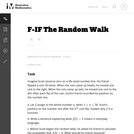Unrestricted Use
CC BY
Rating
0.0 stars

This task requires interpreting a function in a non-standard context. While the domain and range of this function are both numbers, the way in which the function is determined is not via a formula but by a (pre-determined) sequence of coin flips. In addition, the task provides an opportunity to compute some probabilities in a discrete situation.

Subject:
Functions
Mathematics
Material Type:
Activity/Lab
Provider:
Illustrative Mathematics
Provider Set:
Illustrative Mathematics
Author:
Illustrative Mathematics
05/01/2012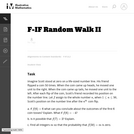Unrestricted Use
CC BY
Rating
0.0 stars

The task is better suited for instruction than for assessment as it provides students with a non standard setting in which to interpret the meaning of functions. Students should carry out the process of flipping a coin and modeling this Random Walk in order to develop a sense of the process before analyzing it mathematically.

Subject:
Functions
Mathematics
Material Type:
Activity/Lab
Provider:
Illustrative Mathematics
Provider Set:
Illustrative Mathematics
Author:
Illustrative Mathematics
06/06/2012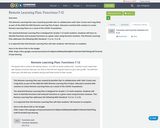Conditional Remix & Share Permitted
CC BY-NC-SA
Rating
0.0 stars

This Remote Learning Plan was created by Jennifer Gier in collaboration with Tyler Cronin and Craig Hicks as part of the 2020 ESU-NDE Remote Learning Plan Project. Educators worked with coaches to create Remote Learning Plans as a result of the COVID-19 pandemic.The attached Remote Learning Plan is designed for Grades 7-12 math&nbsp;students. Students will learn to identify functions and evaluate functions at a given value using function notation. This Remote Learning Plan addresses the following NDE Standard: 11.2.1a, 11.2.1bIt is expected that this Remote Learning Plan will take students 140 minutes to complete.Here is the direct link to the Google Slide:&nbsp;https://docs.google.com/presentation/d/1eBIpImvKMWaAZNwdJMH7chKmAxYGGFVlHCgm2kT3rc0/edit?usp=sharing&nbsp;

Subject:
Mathematics
Material Type:
Activity/Lab
Homework/Assignment
Interactive
Lesson
Author:
Jenny Gier
07/22/2020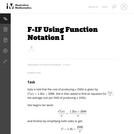Unrestricted Use
CC BY
Rating
0.0 stars

This task deals with a student error that may occur while students are completing F-IF Average Cost.

Subject:
Functions
Mathematics
Material Type:
Activity/Lab
Provider:
Illustrative Mathematics
Provider Set:
Illustrative Mathematics
Author:
Illustrative Mathematics
05/01/2012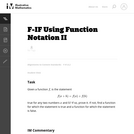Unrestricted Use
CC BY
Rating
0.0 stars

The purpose of the task is to explicitly identify a common error made by many students, when they make use of the "identity" f(x+h)=f(x)+f(h). A function f cannot in general be distributed over a sum of inputs.

Subject:
Functions
Mathematics
Material Type:
Activity/Lab
Provider:
Illustrative Mathematics
Provider Set:
Illustrative Mathematics
Author:
Illustrative Mathematics
05/01/2012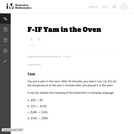Unrestricted Use
CC BY
Rating
0.0 stars

The purpose of this task is to give students practice interpreting statements using function notation. It can be used as a diagnostic if students seem to be having trouble with function notation, for example interpreting f(x) as the product of f and x.

Subject:
Functions
Mathematics
Material Type:
Activity/Lab
Provider:
Illustrative Mathematics
Provider Set:
Illustrative Mathematics
Author:
Illustrative Mathematics
05/01/2012Unrestricted Use
CC BY
Rating
0.0 stars

This is a simple task touching on two key points of functions. First, there is the idea that not all functions have real numbers as domain and range values. Second, the task addresses the issue of when a function admits an inverse, and the process of "restricting the domain" in order to achieve an invertible function.

Subject:
Functions
Mathematics
Material Type:
Activity/Lab
Provider:
Illustrative Mathematics
Provider Set:
Illustrative Mathematics
Author:
Illustrative Mathematics